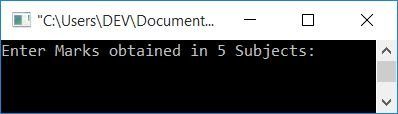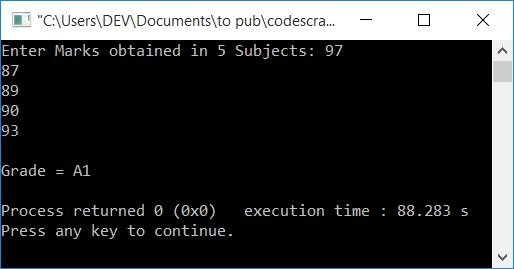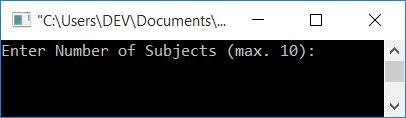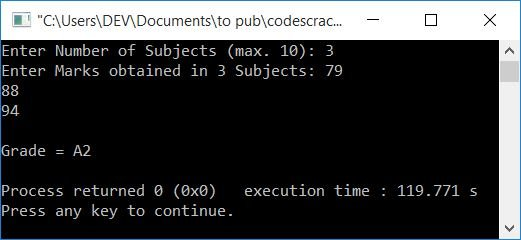# C++ program to calculate the grade of a student

Here you will learn and get code for finding the grade of a student based on marks obtained in C++. There are two programs you'll go through:

The grade must be calculated based on the following pattern:

91-100 A1
81-90 A2
71-80 B1
61-70 B2
51-60 C1
41-50 C2
33-40 D
21-32 E1
0-20 E2

## Calculate the Grade of the Student in C++

To calculate the grade of a student on the basis of total marks in C++ programming, you have to ask the user to enter marks obtained in five subjects. To calculate the average mark, add the marks from all five subjects and divide them by five. And based on this average mark, find the grade as per the table given above:

```#include<iostream>
using namespace std;
int main()
{
int i;
float mark, sum=0, avg;
cout<<"Enter Marks obtained in 5 Subjects: ";
for(i=0; i<5; i++)
{
cin>>mark;
sum = sum+mark;
}
avg = sum/5;
if(avg>=91 && avg<=100)
cout<<"A1";
else if(avg>=81 && avg<91)
cout<<"A2";
else if(avg>=71 && avg<81)
cout<<"B1";
else if(avg>=61 && avg<71)
cout<<"B2";
else if(avg>=51 && avg<61)
cout<<"C1";
else if(avg>=41 && avg<51)
cout<<"C2";
else if(avg>=33 && avg<41)
cout<<"D";
else if(avg>=21 && avg<33)
cout<<"E1";
else if(avg>=0 && avg<21)
cout<<"E2";
else
cout<<"Invalid!";
cout<<endl;
return 0;
}```

This program was built and runs under the Code::Blocks IDE. Here is its sample run:Now supply the marks obtained in 5 subjects, say 97, 87, 89, 90, and 93, and press the ENTER key to see the grade based on these marks as shown in the output given below:### Using a user-defined Function

This program does the same job as the previous one, which is to find and print the grade of the student. But an extra feature was added to it. The extra feature is that the user can enter the subject's number. For example, if he/she enters 3 as the number of subjects, the program will then ask for the marks obtained in those three subjects.

This program calculates the grade of a student using a user-defined function, findGrade(). This function takes two arguments: the first one is an array that holds all the marks entered by the user, and the second is size (that is, the number of subjects). It returns a string-type value. To learn more about function in C++, refer to its separate tutorial.

```#include<iostream>
using namespace std;
int main()
{
int i, n;
float mark;
cout<<"Enter Number of Subjects (max. 10): ";
cin>>n;
cout<<"Enter Marks obtained in "<<n<<" Subjects: ";
for(i=0; i<n; i++)
cin>>mark[i];
cout<<endl;
return 0;
}
const char* findGrade(float mark[], int n)
{
int i;
float sum=0, avg;
for(i=0; i<n; i++)
sum = sum+mark[i];
avg = sum/n;
if(avg>=91 && avg<=100)
return "A1";
else if(avg>=81 && avg<91)
return "A2";
else if(avg>=71 && avg<81)
return "B1";
else if(avg>=61 && avg<71)
return "B2";
else if(avg>=51 && avg<61)
return "C1";
else if(avg>=41 && avg<51)
return "C2";
else if(avg>=33 && avg<41)
return "D";
else if(avg>=21 && avg<33)
return "E1";
else if(avg>=0 && avg<21)
return "E2";
else
return "Invalid!";
}```

Below is the initial snapshot of the sample run:Now enter the number of subjects, say 3, and then enter the marks obtained in those three subjects to find the grade as shown in the output given below:#### Same Program in Other Languages

C++ Online Test

« Previous Program Next Program »